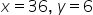Mathematics
Easy

Question

# The father's age is six times his son's age. Four years hence, the age of the father will be four times his son's age. The present ages, in years, of the son and the father respectively are

## 4 and 245 and 306 and 36 3 and 24Hint:

## The correct answer is: 6 and 36

### Here we have to find that age of father and son,Firstly , it is given at first the father age is six times its son .Lets father be a and son be b,So , b = 6a ---(1)And after four year ,Father age is four times to his son , so we can write, b+4 = 4(a+4) [we add 4 in both sides because it is after 4 years so both age ages are added by 4] b + 4 =4a + 16 6a + 4 = 4a + 16 ( using (1)) 6a – 4a = 16 – 4 2a =12 a = 6 put value of a in eq (1),b = 6ab = 6 x 6b = 36Therefore , The present age of son and father is 6 and 36The correct answer is 6 and 36.Or,Let, the present age of father = x yearsLet, the present age of son = y yearsGiven that fathers Age is 6 time of sons age⇒x=6y…(1)After four years,Age of the father will be four times of his son’s age⇒x+4=4(y+4)⇒x+4=4y+16⇒x=4y+16−4⇒x=4y+12…(2) Step2: Solving the equations.Substitute equation (1) in equation (2)⇒6y=4y+12⇒2y=12⇒y=6Substitute y=6 in equation (1)⇒x=36Hence, the solution of the father and his son ages are.

In this question, we have to make equation on the given date about ages. Consider all the character in similar ages question be some variable and make the equation on given details.

### Related Questions to study#### With Turito Foundation.#### Get an Expert Advice From Turito.